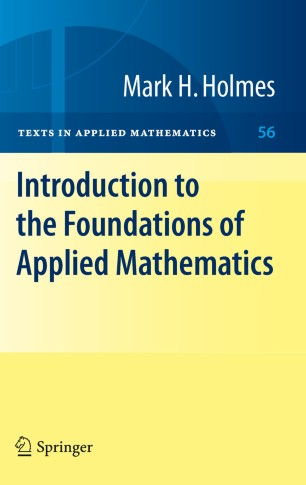# Introduction to the Foundations of Applied Mathematics

• Mark H. HolmesTextbook

Part of the Texts in Applied Mathematics book series (TAM, volume 56)

1. Front Matter
Pages i-xiv
2. Mark H. Holmes
Pages 1-42
3. Mark H. Holmes
Pages 43-85
4. Mark H. Holmes
Pages 87-140
5. Mark H. Holmes
Pages 141-203
6. Mark H. Holmes
Pages 205-264
7. Mark H. Holmes
Pages 265-310
8. Mark H. Holmes
Pages 311-349
9. Mark H. Holmes
Pages 351-401
10. Mark H. Holmes
Pages 403-440
11. Back Matter
Pages 441-468

### Introduction

The objective of this textbook is the construction, analysis, and interpretation of mathematical models to help us understand the world we live in. Rather than follow a case study approach it develops the mathematical and physical ideas that are fundamental in understanding contemporary problems in science and engineering. Science evolves, and this means that the problems of current interest continually change.

What does not change as quickly is the approach used to derive the relevant mathematical models, and the methods used to analyze the models. Consequently, this book is written in such a way as to establish the mathematical ideas underlying model development independently of a specific application. This does not mean applications are not considered, they are, and connections with experiment are a staple of this book.

The book, as well as the individual chapters, is written in such a way that the material becomes more sophisticated as you progress. This provides some flexibility in how the book is used, allowing consideration for the breadth and depth of the material covered.

Moreover, there are a wide spectrum of exercises and detailed illustrations that significantly enrich the material. Students and researchers interested in mathematical modelling in mathematics, physics, engineering and the applied sciences will find this text useful.

### Keywords

Applied Mathematics Foundations Identity Introduction calculus equation linear optimization mathematics theorem

#### Authors and affiliations

• Mark H. Holmes
• 1
1. 1.Academic Science of the Material, Science and EngineeringRensselaer Polytechnic InstituteTroyU.S.A.

### Bibliographic information

• DOI https://doi.org/10.1007/978-0-387-87765-5